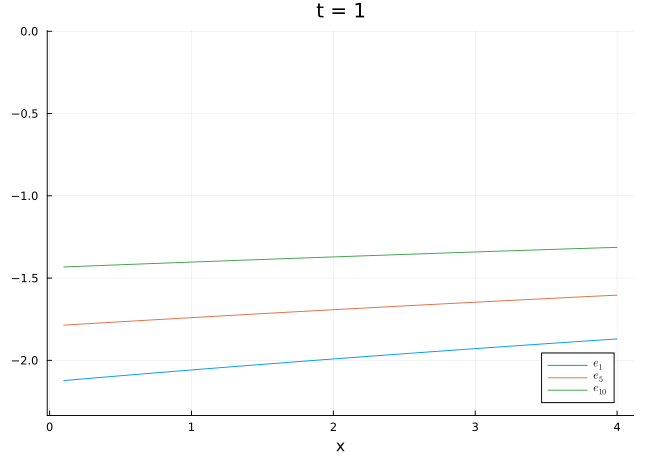# Scientific Communication with Quarto: Economic Models

Julia
Observable JS
Quarto
Author

Kazuharu Yanagimoto

Published

June 10, 2023

## Quarto Is not Just for Static Reports

Notebook coding (Jupyter, Rmarkdown, and Quarto) has gotten popular in the last decade. It allows us to tell a story with code and data, and it makes scientific communication more transparent and reproducible. I use Quarto to share my current results with my supervisor and coauthors, especially when I am in the playground stage of the project.

While it is naturally useful in that it is an easy tool for creating reports, exploiting its HTML feature, we can enrich communication even further. Here, I introduce my use case of GIF and Observable JS for economic models with Julia.

Imagine that you are working on a very simple life-cycle model:

For $$t = 1, \dots, T$$, households solve $V(t, e, x) = \max_{c, x'} \frac{c^{1 - \sigma}}{1 - \sigma} + \beta \mathbb{E}V(t + 1, e', x')$ \begin{aligned} c + x' &\le (1 + r)x + ew \\ \text{Pr}(e' | e) &= \Gamma(e) \\ c, x' &\ge 0 \end{aligned}

See the following code for the parameters and the solution algorithm.

Code
using Parameters
using QuantEcon
using Plots
using LaTeXStrings
using CSV
using DataFrames

@with_kw struct Model

# Grid for x
nₓ::Int = 30
x̲::Float64 = 0.1
x̄::Float64 = 4.0
x_grid::Vector{Float64} = range(start = x̲, stop = x̄, length = nₓ)

# Grid for e: parameters for Tauchen
nₑ::Int = 10
σ_ε::Float64 = 0.02058
λ_ε::Float64 = 0.99
m::Float64 = 1.5
mc::MarkovChain = tauchen(nₑ, λ_ε, σ_ε, 0.0, m)
e_grid::Vector{Float64} = exp.(mc.state_values)
P::Matrix{Float64} = mc.p

# Utility function
σ::Float64 = 2.0
β::Float64 = 0.97
T::Int = 10

# Prices
r::Float64 = 0.07
w::Float64 = 5.0

end

u(c, m::Model) = isone(m.σ) ? log(c) : c^(1 - m.σ) / (1 - m.σ);

function solve(m::Model)

@unpack T, nₓ, nₑ, r, w, β, P, x_grid, e_grid = m

V = zeros(nₓ, nₑ, T)

for t = T:-1:1, iₓ= 1:nₓ, iₑ = 1:nₑ

utility = -Inf
for iₓ′ = 1:nₓ

expected = (t == T) ? 0.0 :
sum(P[iₑ, iₑ′] * V[iₓ′, iₑ′, t+1] for iₑ′ = 1:nₑ)
c = (1 + r) * x_grid[iₓ] + e_grid[iₑ] * w - x_grid[iₓ′]

if c > 0
utility = max(u(c, m) + β * expected, utility)
end

end

V[iₓ, iₑ, t] = utility
end

return V
end

m = Model()
V = solve(m);

A traditional way to show the value function is to plot for selected $$e$$ and $$t$$.

Code
ps = []

y̲, ȳ = minimum(V) * 1.1, maximum(V) + 0.1
for (i, t) ∈ enumerate([1, 4, 7, 10])

p = plot(m.x_grid, V[:, 1, t],
xlabel = "x",
ylims = (y̲, ȳ),
label = L"e_1",
legend = :bottomright,
title = "t = $t") plot!(m.x_grid, V[:, 5, t], label = L"e_5") plot!(m.x_grid, V[:, 10, t], label = L"e_{10}") push!(ps, p) end plot(ps...) This is not bad, however, we can add more information by using GIF. ## GIF While this is not so famous, we can create a GIF animation just by adding @animate macro in Julia. Code anim = @animate for t = 1:10 plot(m.x_grid, V[:, 1, t], xlabel = "x", ylims = (y̲, ȳ), label = L"e_1", legend = :bottomright, title = "t =$t")
plot!(m.x_grid, V[:, 5, t], label = L"e_5")
plot!(m.x_grid, V[:, 10, t], label = L"e_{10}")
end

gif(anim, "img/anim.gif", fps = 1)See? This is very intuitive and informative! But if you are interested in the changes with multiple parameters, you may want to try Observable JS.

## Observable JS

Observable is a JavaScript notebook that allows us to create interactive visualizations. It is free and open-source, and we can embed it in our Quarto document. Since the Observable is JavaScript-based, we can put an interactive (dynamic) visualization in static HTML documents!

For example, you are now interested in the changes in the value function with $$r$$ and $$w$$. To implement it in Observable, we first need to create a CSV file1 that contains the results of the simulation.

Code
function solve_partial(r, w)
m = Model(r = r, w = w)
V = solve(m)
return [
(x = x, ie = iₑ, t = t, V = V[iₓ, iₑ, t], r = m.r, w = m.w)
for (iₓ, x) ∈ enumerate(m.x_grid)
for (iₑ, e) ∈ enumerate(m.e_grid) if iₑ ∈ [1, 5, 10]
for t ∈ 1:m.T
]
end

res = Iterators.flatten([solve_partial(r, w) for r ∈ 0.01:0.02:0.15 for w ∈ 2.0:0.5:10.0])
df = DataFrame(res)
d = Dict(1 => "e₁", 5 => "e₅", 10 => "e₁₀")
df.lbl = [d[i] for i in df.ie]
CSV.write("data.csv", df);

This CSV file is then loaded in Observable and filtered by the parameters.2

Code
data = FileAttachment("data.csv").csv()
filtered = data.filter(function(sim) {
return sim.r == r && sim.w == w && sim.t == t
})

Adding sliders and a plot, we have a cool interactive visualization!

Code
viewof t = Inputs.range(
[1, 10],
{value: 1, step: 1, label: "t"}
)
viewof r = Inputs.range(
[0.01, 0.15],
{value: 0.07, step: 0.02, label: "r"}
)
viewof w = Inputs.range(
[2.0, 10.0],
{value: 5.0, step: 0.5, label: "w"}
)
Code
Plot.plot({
marginLeft: 50,
height: 400,
color: {domain: ["e₁", "e₅", "e₁₀"], legend: true},
x: {domain: [0.0, 4.0]},
y: {domain: [-5.5, 0.0]},
marks: [
Plot.lineY(filtered, {x: "x", y: "V", stroke: "lbl"}),
]
})

Happy Quarto life 🥂!

## Footnotes

1. You can use CSV, TSV, JSON, Arrow (uncompressed), and SQLite as data input. See Data Sources↩︎

2. While the parameters r, w, and t are defined in the next cell, the order of the Observable JS cells do not change the results.↩︎